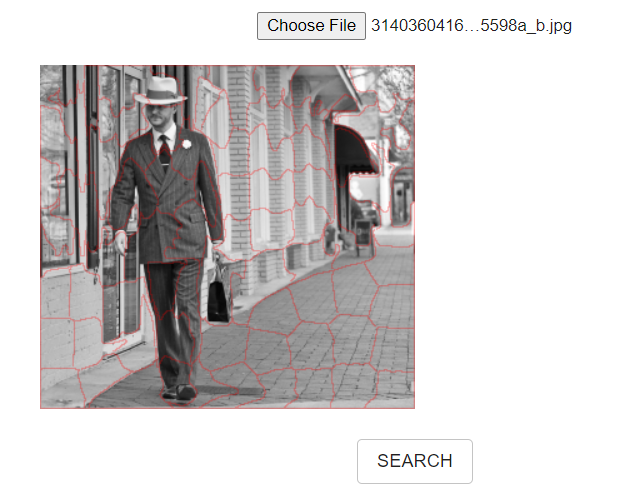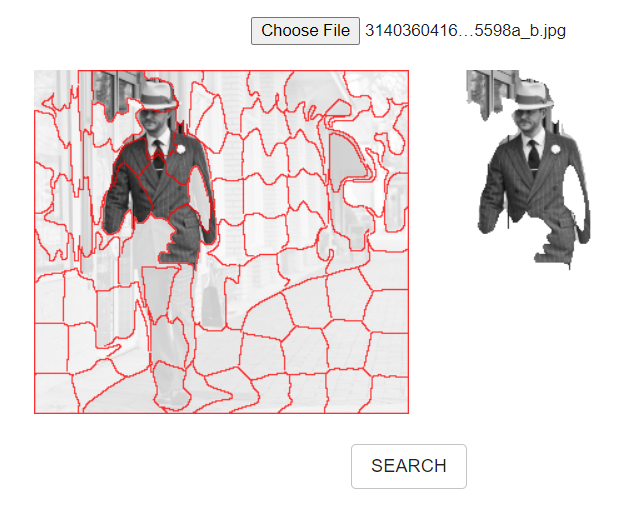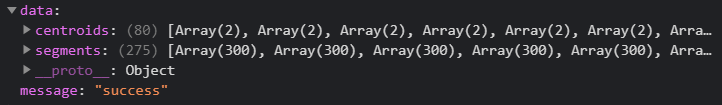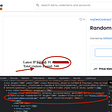# Intention and Background

There are time when we want to search similar image, the search algorithm would not know the “attention” from the crowded information in input image.

So I am thinking to have a web interface to allow user to select portion of image as search input.

# What it does

User load an image (appear on the left), and then generate superpixels and user can click on those superpixels to “select” them, and the selected portion would be display on the right.

Upon clicking search button, the “selected portion” would be used to search for similar images and display a sliding drawer (from left)

Upon clicking the search result, a pop up would be loaded with some metadata.

# What is superpixel?

Consider it as a grouping of similar pixels as a “superpixel”, better explanation from this article:

# Solution architecture and framework used

The solution include a React.js built frontend and a FastAPI backend which perform the superpixel.

# Backend (for calculate superpixels)

For simplicity on prototyping, the superpixel generation is using skimage’s SLIC implementation, and this is the reason why I would use a backend API as skimage is written in Python and my frontend is on JavaScript.

The main Python class is as below:

`import ioimport sysimport base64import tracebackimport numpy as npimport skimageimport skimage.ioimport skimage.segmentationfrom skimage.measure import regionpropsclass ImageProcessor:  def __init__(self):    passdef convert_image_b64string_to_ndarray(self, base64_image):    encoded = base64.b64decode(base64_image)    image_array = skimage.io.imread(io.BytesIO(encoded))[:,:,:3]    return image_arraydef produce_segments(self, image_array, n_segments=10, sigma=3):    try:      segments = skimage.segmentation.slic(image_array, n_segments = n_segments, sigma = sigma)      regions = regionprops(segments+1)      centroids = [props.centroid for props in regions]      return {          "segments": segments,          "centroids": centroids      }    except Exception as err:      traceback.print_exception(*sys.exc_info())def __call__(self, base64_image, n_segments=10, sigma=3):    img_array = self.convert_image_b64string_to_ndarray(base64_image)    result = self.produce_segments(img_array, n_segments, sigma)    return result`

Note that because it’s an API, I decided to have the API input as base64 encoded image, so there is this supporting function to transform the image into input required by skimage’s SLIC:

`def convert_image_b64string_to_ndarray(self, base64_image):    encoded = base64.b64decode(base64_image)    image_array = skimage.io.imread(io.BytesIO(encoded))[:,:,:3]    return image_array`

The skimage.io.imread would return Numpy array of (Width, Height, Channel), for SLIC, it does support RGB or RGBA, but for simplicity, I decided to drop alpha channel by slicing the last dimension with [:, :, :3].

# Frontend

For having the user experience of overlaying the superpixels grid over the original image and mouse over effect like below:Mouse not over, superpixels boundary line color lighter to see more clear on original imageMouse over with some superpixel being selected, superpixels boundary line color darken to make it easy to see as well as the unselected pixel in original image would dim

I have the canvas components like follow:

I chose tensorflow.js because I would love to flex my muscles on using more machine learning tools, and I “believe” it also benefit from able to leverage WebGL (correct me if I am wrong please).

The high level flow as follow:

`1. Load the image, bind it to image canvas to display , as well as load the image as tensorflow.js tensor2. Send the image to generate superpixel (with backend API), bind the result superpixel and draw the boundary of superpixels on superpixel canvas3. Upon click of superpixel area on superpixel canvas,  check the area occupied by the superpixels, and maintain a mask (tensorflow.js tensor) for result display`

We use a file type input to allow user to browse an image and then use a FileReader to load the image into base64 string, and finally remove the data url prefix.

`var base64Result;var dataUrlStripped;// fileInput is a <input type="file"> controlconst fileToUpload = fileInput.current.files;const fileReader = new FileReader();fileReader.onloadend = async () => { base64Result = fileReader.result; // remove the prefix / type info from the data // e.g. "data:image/png;base64,iVBORw0KGgoAAAANSUhEU..." // stripped => iVBORw0KGgoAAAANSUhEU... dataUrlStripped = base64Result.split(",")?.;}fileReader.readAsDataURL(fileToUpload);`

Put the image to canvas and then store in tensorflow.js tensor

Here we create a Image, and upon load the image from the dataUrl data, we draw it to the canvas context and load it into tensorflow.js tensor.

`// get the context from the <canvas>const ctx = canvasRef.getContext("2d");// prepare an image for loading const image = new Image(); image.onload = function(){ ctx.canvas.width = maxWidth; ctx.canvas.height = image.height*maxWidth/image.width; ctx.drawImage(image, 0, 0, image.width, image.height, 0, 0, ctx.canvas.width, ctx.canvas.height);const imageTensor = tf.browser.fromPixels(ctx); const canvasBoundingRect = canvasRef.getBoundingClientRect(); const canvasBase64 = canvasRef.toDataURL();};image.src = dataUrlStripped;`

Note that the tf.browser.fromPixels support multiple input type, the reason I choose to load it from canvas because I am using a resized version of the image.

From the API return we got the number of centroids and per pixel superpixel ID (imply which cluster the pixel belongs to)This is the expected return from the Python API, and the segment is 275 x 300, which is correlated to image size (300 x 275)

Rendering the pixel and maintaining a mask for selected superpixels

The core function to prepare tensorflow.js tensor of the segments and prepare the rendering of drawing superpixel boundary is as follow (we would discuss this code block in 3 parts below):

`function prepareTfSegments(segmentTensor){ return tf.tidy(()=>{  const inputMax = segmentTensor.max();  const inputMin = segmentTensor.min();  // (value - min) / (max - min) * 255  const normalizedInputs = segmentTensor.sub(inputMin).div(inputMax.sub(inputMin)).mul(tf.scalar(255));  // stack 3 times, then add alpha  let expandedInputs = normalizedInputs;  expandedInputs = expandedInputs.expandDims(2); // h,w => h,w,1  expandedInputs = tf.tile(expandedInputs, [1, 1, 3]); // repeat at last dim (channel) | h,w,1 => h,w,3  const alpha = tf.onesLike(normalizedInputs).mul(tf.scalar(255)).expandDims(2); // h,w => h,w,1  expandedInputs = tf.concat([expandedInputs,alpha], 2); // h,w,4// edge detection  const kernel = tf.tensor([   [-1,-1,-1],   [-1, 8,-1],   [-1,-1,-1]  ]).expandDims(2).expandDims(3);const [imgH, imgW] = normalizedInputs.shape  const model = tf.sequential({   layers: [tf.layers.conv2d({kernelSize:3, filters:1, strides:1, useBias:false, padding:"same", inputShape: [imgH, imgW, 1], weights:[kernel]})]  });const edgeResult = model.predict(normalizedInputs.expandDims(2).expandDims(0)); // h,w => 1, h, w, 1let edgeMask = tf.greater(edgeResult.toInt(), tf.scalar(0)).mul(tf.scalar(255)).toInt().squeeze(0); // 1, h, w, 1 => h, w, 1  const zeroChannels = tf.zerosLike(normalizedInputs).expandDims(2); // h,w => h,w,1  edgeMask = tf.concat([edgeMask,zeroChannels,zeroChannels,edgeMask], 2);// BOTH h,w,4  return [expandedInputs, edgeMask]; });}`

Note that the code is wrap inside tf.tidy(), this is the function that help tensorflow.js to clean up any temporary tensor in memory throughout calculation.

Explaining the first part — normalize / stretch the input range

Because our segmentTensor is having values of superpixel ID, let say we have 30 superpixels, we would have values between 1 to 30.

Example below is a height = 3, width = 4 image with 5 superpixels:

` 1 | 1 | 2 | 2  1 | 1 | 3 | 3 4 | 5 | 5 | 3`

For better distinguish between region of superpixel and draw the boundary (with edge detection, see third part below), an edge detection kernel is being use and I would like to stretch the value from 1–30 to 1–255.

`const inputMax = segmentTensor.max();const inputMin = segmentTensor.min();// (value - min) / (max - min) * 255const normalizedInputs = segmentTensor.sub(inputMin).div(inputMax.sub(inputMin)).mul(tf.scalar(255));`

The tensorflow.js API, does not have operator overload, so to perform x-y, we would do:

`x.sub(y)`

To operate the tensor with a scalar, one would need to create a tensorflow scalar by tf.scalar(value), as tensorflow.js cannot operate a JavaScript number directly with tensor.

Explaining the second part — expand the input from 1 channel to 4 channels

Given our superpixel is a tensor of shape [h,w], and my target is a canvas that overlay on top of the image, so I would prefer to leverage alpha channel. This require expanding the channel (which the 4 channels would be R, G, B, alpha).

`// stack 3 times, then add alphalet expandedInputs = normalizedInputs;expandedInputs = expandedInputs.expandDims(2); expandedInputs = tf.tile(expandedInputs, [1, 1, 3]); // repeat at last dim (channel) | h,w,1 => h,w,3const alpha = tf.onesLike(normalizedInputs).mul(tf.scalar(255)).expandDims(2); // h,w => h,w,1expandedInputs = tf.concat([expandedInputs,alpha], 2); // h,w,4`

In tensorflow.js, tensor.expandDims(axis) , which axis count from 0, the function would add a dimension at particular axis, the original dimension is [h, w], and we want to first add one more dimension at 2 and make it become [h,w,1]

The tf.tile(tensor, repeatPerDimension) is used to expand the last dimension to 3, these 3 dimensions are for the R,G,B channels.

Then we add alpha channel which is all 255, the operation is to create tensor which have same dimension as original tensor [h, w] with tf.onesLike(tensor), then multiple by scalar 255, and finally make it [h,w,1] througt expandDims so in next step we can concatenate.

Final operation is to use tf.concat to merge the tensor of RGB channel [h,w,3] with tensor of alpha channel [h,w,1] at axis 2 to become [h,w,4] tensor.

Explaining the third part — using an edge detection kernel to detect boundary of superpixels

Note: reviewing this code again now, I feel I am wasting processing power as I can process on single channel tensor instead of the 4 channel verion of segment tensor, but bare with me for now.

First off we make a simple edge detection kernel, this is proven to be enough for my case.

And I leverage the tensorflow.js convolution layer with the customized kernel as weight and no bias, this layer would be used to go over the segment tensor like sliding window process.

Basic operation between edge and kernel:

` 1 | 1 | 1   -1 | -1 | -1 1 | 1 | 1 x -1 |  8 | -1 = 0 1 | 1 | 1   -1 | -1 | -1 1 | 1 | 1   -1 | -1 | -1 1 | 1 | 1 x -1 |  8 | -1 = -2 1 | 2 | 2   -1 | -1 | -1`

For more understanding on Convolution, see this great article:

Because the final target is for making RGBA tensor of edges, I would take the convolution output, make it as boolean, and scale it to 255, this is pass as first channel (red channel) and last channel (alpha channel) and then stuff in green and blue channel with zeros.

Note that I spot something wrong in my code, I use “edgeMask > 0” as boolean calculation, but when it’s an edge, the convolution result might be -ve number see example above)

`// edge detectionconst kernel = tf.tensor([  [-1,-1,-1],  [-1, 8,-1],  [-1,-1,-1]]).expandDims(2).expandDims(3);const [imgH, imgW] = normalizedInputs.shapeconst model = tf.sequential({  layers: [tf.layers.conv2d({kernelSize:3, filters:1, strides:1, useBias:false, padding:"same", inputShape: [imgH, imgW, 1], weights:[kernel]})]});const edgeResult = model.predict(normalizedInputs.expandDims(2).expandDims(0)); // h,w => 1, h, w, 1let edgeMask = tf.greater(edgeResult.toInt(), tf.scalar(0)).mul(tf.scalar(255)).toInt().squeeze(0); // 1, h, w, 1 => h, w, 1const zeroChannels = tf.zerosLike(normalizedInputs).expandDims(2); // h,w => h,w,1edgeMask = tf.concat([edgeMask,zeroChannels,zeroChannels,edgeMask], 2);`The edge is rendered on the canvas above image canvas with red color

Handling on click of superpixel

The behavior is expected to be like this, when user clicks a point inside a superpixel, the display canvas on the right would display the superpixel patch if the superpixel was not clicked before, or erase it if it did.

The idea is as follow:

`0. keep track of a mask of pixels corresponded to selected superpixels1. upon click, find out the superpixel ID at the click (x,y) location, and then find out all pixels that is share the same superpixel ID2. perform a XOR operation between the mask and the result from point 13. use the updated mask from 2 above and the image tensor to generate the masked image correspond to the mask (i.e. selected superpixels) and render it`

Knowing the superpixel ID value of the clicked location

The source is the segment tensor in shape of [h,w,1] (backend API return before we process it into RGBA 4 channel tensor), the function call to access the tensor value that I know of from tensorflow.js is tf.slice(…).

`tf.slice(canvasTensor.current,[y,x,0],[1,1,1]).data().then((v) => {  const value = v;  // this is superpixel ID of the x,y location  ...}`

XOR operation

`function calculateMask(mask, segment, valueSelected){ return tf.tidy(()=>{  const valueHitMask = segment.equal(tf.scalar(valueSelected));  return mask.logicalXor(valueHitMask); });}`

Note that the mask is a cumulative mask tensor we would use to indicate what’s being selected or not, the segment is the [h,w] of segment ID of each pixel and the valueSelected is the value of mouse clicked point at x,y.

Generate result image that’s masked (that only show the selected superpixels)

Finally, we take the mask tensor and apply it on the image tensor.

`const canvasTensor = imageTensor.mul(maskTensor.toInt()).add(maskTensor.logicalNot().toInt().mul(tf.scalar(255)));tf.browser.toPixels(canvasTensor.toInt(), canvasRef);`

The calculation translate into:

`(image tensor * mask) + (!mask * 255)`

Reason for all those toInt() is converting boolean into integer, as tensorflow.js seems does not support implicit conversion during calculation between boolean and integer tensors.

The “!mask * 255” part basically add background as “white” (when RGB channels are all 255).

And voilà~

# Final thoughts

Thank you for reading till the end, it probably took you some headache to read through. Some of the code is simplified (without testing the simplified version) to facilitate explanation.

When I review the code again, I feel it can be rewritten in a more efficient way for some part, or even found bugs. Feel free to points out what I did wrong or any better ways to implement.

--

--

--

Love podcasts or audiobooks? Learn on the go with our new app.

## Connecting to GitLab with SSH## wtfCTF 2022— web challenge [1–4] WalkThrough## How I learnt to love and hate Terraform in the past few weeks## Dollaremon Swap launch!!## PortSwigger Lab: Modifying serialized data types | WalkThrough## Here Are 6 Front-End Challenges to Code## Stale braches cleanup in Git repo## Avalanche : Gas too low — code”:-32603,”data”:{“code”:-32000,”message”:”intrinsic gas too low”## How To Perform Rapid Scan v1.2 Using Kali Linux## Diffie-Hellman Key Exchange## How OpenSea allows Cross-Site-Scripting Attacks (XSS)